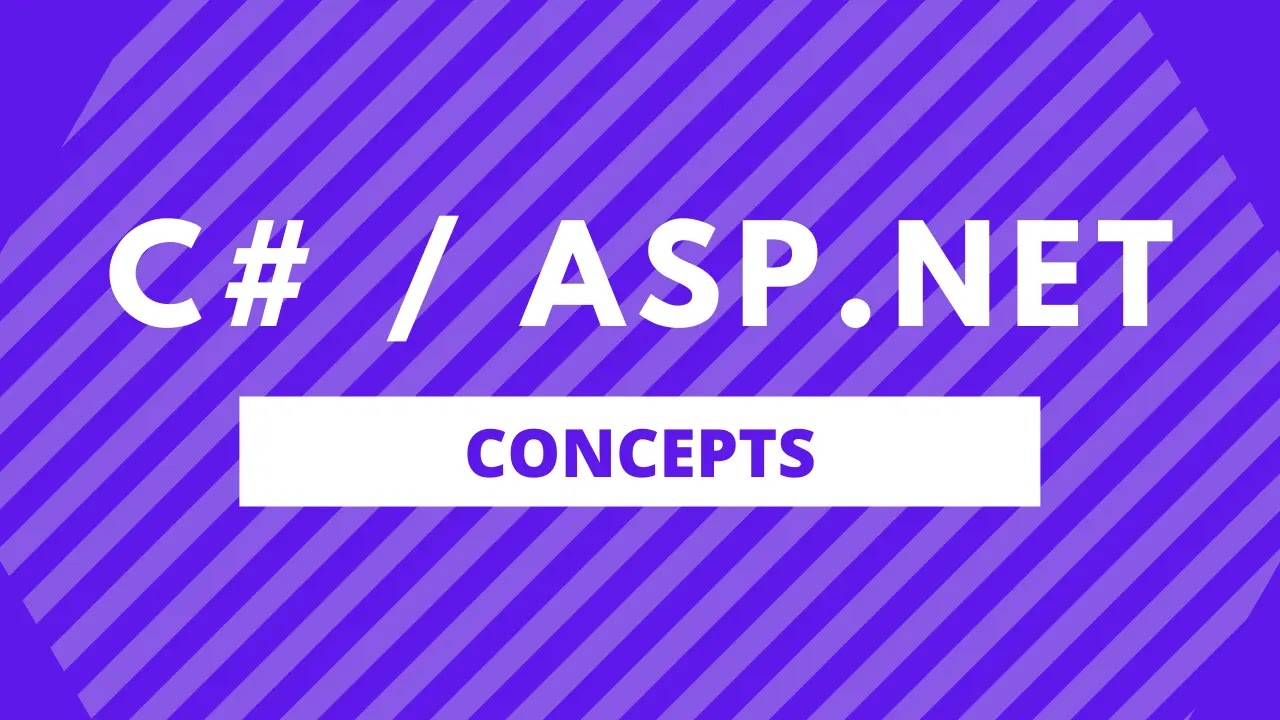# Char Array To String In C#

Sameer Saini April 06, 2022In this blog post, we will learn how to convert a Char Array To String in C#.

A char array is an array of character types. That means they hold a single character value in each of its elements.

Let's say you have a use case in which you want to join the char array to form a string in C#.

In this article, we will see how easy it is to convert a char array to string.

## Convert Char Array To String In C# Using Foreach Loop

In this method, we will convert a char array to string using a foreach loop.

We will iterate through the elements of the char array and concatenate their values together to form a string value.

char[] charArray = new char[] { 'c', 'o', 'd', 'e', ' ', 'l', 'i', 'k', 'e', ' ', 'a', ' ', 'd', 'e', 'v' }; string charString = ""; foreach (char element in charArray) { charString += element; } Console.WriteLine(charString);

Result:

code like a dev

As you can see from the example above, we used a char array, the elements had a few values which if joined together formed "code like a dev".

We then used a foreach loop to add each element of the char array to the string.

Finally, we printed the string on the Console.

## Convert Char Array To String In C# Using String.Join() Method

There is another way in which we can do this conversion of converting our char array to a string.

We will use the string.Join() method to join the char array to string.

char[] charArray = new char[] { 'c', 'o', 'd', 'e', ' ', 'l', 'i', 'k', 'e', ' ', 'a', ' ', 'd', 'e', 'v' }; string charString = string.Join("", charArray); Console.WriteLine(charString);

Result:

code like a dev